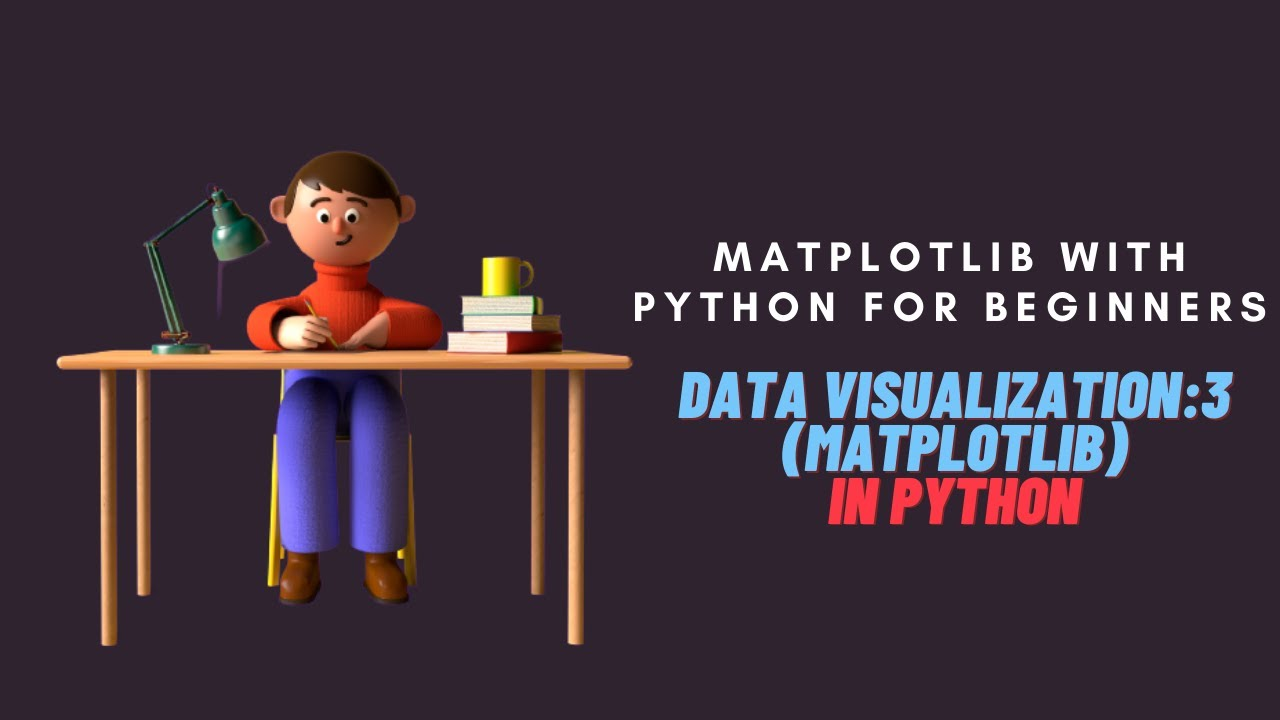# Matplotlib with Python Tutorial for Beginners|Data Visualization with Matplotlib in PythonMatplotlib with Python Tutorial for Beginners | Data Visualization with Matplotlib in Python. This video is a Python Tutorial to learn Python programming with examples. In this video we will see : Matplotlib is an amazing visualization library in Python for 2D plots of arrays. Matplotlib is a multi-platform data visualization library built on NumPy arrays and designed to work with the broader SciPy stack. It was introduced by John Hunter in the year 2002.

This video is a Python Tutorial to learn Python programming with examples

In this video we will see :

• Matplotlib is an amazing visualization library in Python for 2D plots of arrays. Matplotlib is a multi-platform data visualization library built on NumPy arrays and designed to work with the broader SciPy stack. It was introduced by John Hunter in the year 2002.

• The matplotlib is a python 2D plotting library for data visualization and the creation of interactive graphics/ plots. A plot is a graphical representation of data which shows the relationship between two variables or the distribution of data. Subplots are used to display multiple plots in the same window.

Complete Blog on 'Python for Absolute Beginners' - https://seeve.medium.com/python-for-absolute-beginners-d6d967b28552

## top 30 Python Tips and Tricks for Beginners

In this post, we'll learn top 30 Python Tips and Tricks for Beginners